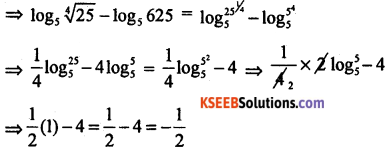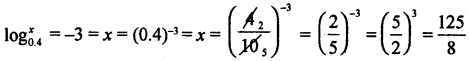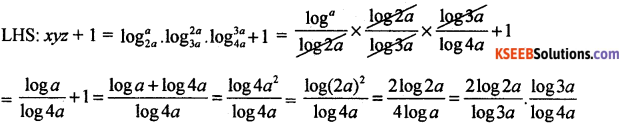# 1st PUC Basic Maths Question Bank Chapter 4 Logarithms

Students can Download Basic Maths Chapter 4 Logarithms Questions and Answers, Notes Pdf, 1st PUC Basic Maths Question Bank with Answers helps you to revise the complete Karnataka State Board Syllabus and score more marks in your examinations.

## Karnataka 1st PUC Basic Maths Question Bank Chapter 4 Logarithms

Logarithms

Question 1.
$$\log _{5} \frac{\sqrt{25}}{625}$$Question 2.
Find x if logx0.4 = -3Question 3.
If x = 27, b = log43 then flow that xb = 64
xb = 27log43
= 33log43 = 3log(4)33
= 3log643 = 64

Question 4.
If log10$$\sqrt{1+x}+3$$ log10 $$\sqrt{1-x}$$ = log10 $$\sqrt{1-x^{2}}+2$$ . Find x.
log10 (x + 1)1/2 + log10(1 – x)3/2 = log10(1 – x2)1/2 + log10010
⇒ (x + 1)1/2 (1 – x)3/2 = (1 – x2)1/2 – 100
⇒ $$\sqrt{1-x^{2}}$$ (1 – x – 100) = 0
⇒ x = ±1. x = -99 But x ≠ ± 1, -99
∴ There is no value of x exists.

Question 5.
If x = loga2a, y = log2a3a, z = log3a4a. Show that xyz + 1 = 2yz.